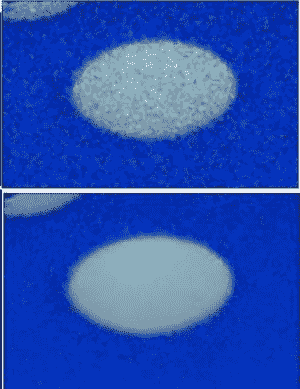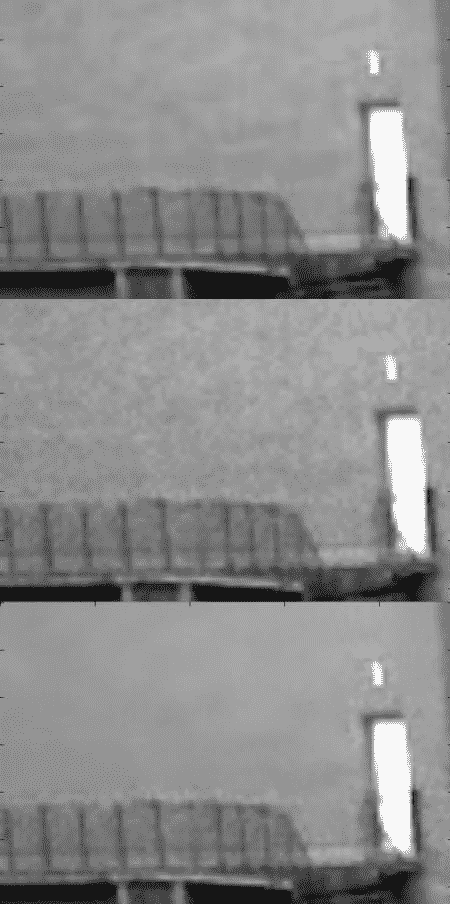# OpenCV-Python 图像去噪

• 您将了解非局部均值去噪算法以消除图像中的噪声。
• 你会看到不同的功能，如 cv.fastNlMeansDenoising（）cv.fastNlMeansDenoisingColored（） 等。

## 图像去噪理论## OpenCV 中的图像去噪

OpenCV 提供了这种技术的四种变体。

1. cv.fastNlMeansDenoising（） – 适用于单个灰度图像
2. cv.fastNlMeansDenoisingColored（） – 适用于彩色图像。
3. cv.fastNlMeansDenoisingMulti（） – 适用于短时间内拍摄的图像序列（灰度图像）
4. cv.fastNlMeansDenoisingColoredMulti（） – 与上述相同，但适用于彩色图像。

• h：参数决定滤波器强度。较高的 h 值可以更好地消除噪声，但也会删除图像的细节。 （10 个没问题）
• hForColorComponents：与 h 相同，但仅适用于彩色图像。 （通常与 h 相同）
• templateWindowSize：应该是奇数。 （推荐 7）
• searchWindowSize：应该是奇数。 （推荐 21）

1. cv.fastNlMeansDenoisingColored（）

import numpy as np
import cv2 as cv
from matplotlib import pyplot as plt

dst=cv.fastNlMeansDenoisingColored(img,None,10,10,7,21)

plt.subplot(121),plt.imshow(img)
plt.subplot(122),plt.imshow(dst)
plt.show()2. cv.fastNlMeansDenoisingMulti（）

import numpy as np
import cv2 as cv
from matplotlib import pyplot as plt

cap = cv.VideoCapture('vtest.avi')
# create a list of first 5 frames
img = [cap.read() for i in xrange(5)]

# convert all to grayscale
gray = [cv.cvtColor(i, cv.COLOR_BGR2GRAY) for i in img]

# convert all to float64
gray = [np.float64(i) for i in gray]

# create a noise of variance 25
noise = np.random.randn(*gray.shape)*10

# Add this noise to images
noisy = [i+noise for i in gray]

# Convert back to uint8
noisy = [np.uint8(np.clip(i,0,255)) for i in noisy]

# Denoise 3rd frame considering all the 5 frames

dst = cv.fastNlMeansDenoisingMulti(noisy, 2, 5, None, 4, 7,35)

plt.subplot(131),plt.imshow(gray,'gray')
plt.subplot(132),plt.imshow(noisy,'gray')
plt.subplot(133),plt.imshow(dst,'gray')
plt.show()• 微信订阅
• 回顶